# Introduction to Java Script Java Script is the

• Slides: 18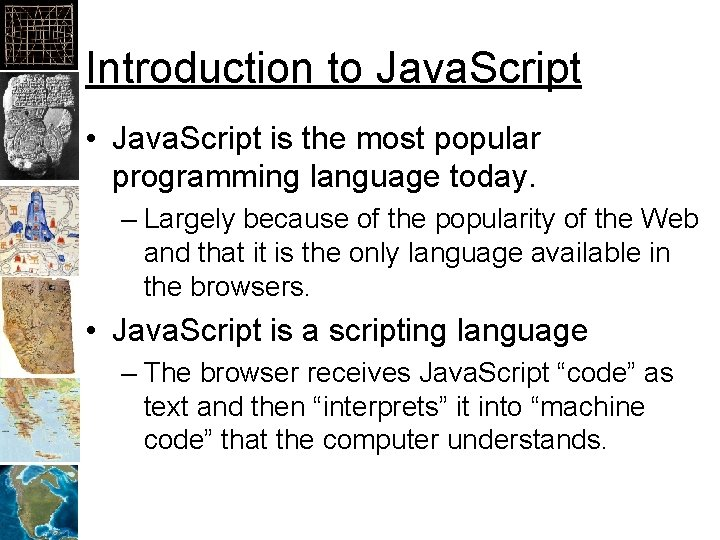Introduction to Java. Script • Java. Script is the most popular programming language today. – Largely because of the popularity of the Web and that it is the only language available in the browsers. • Java. Script is a scripting language – The browser receives Java. Script “code” as text and then “interprets” it into “machine code” that the computer understands.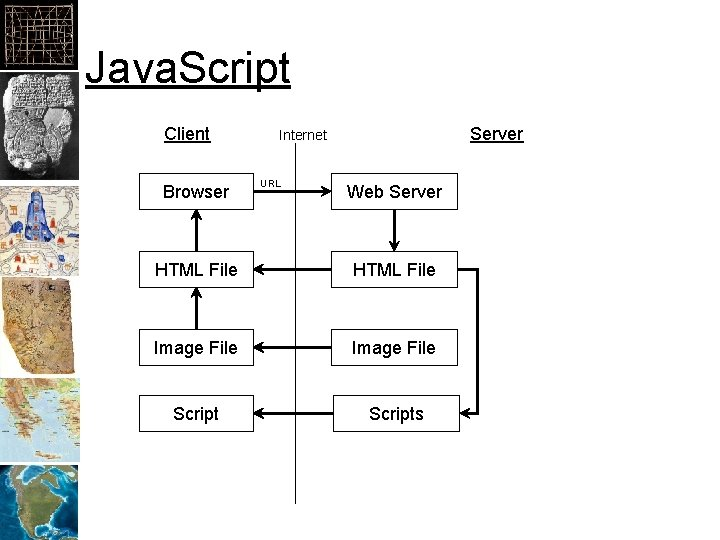Java. Script Client Browser Server Internet URL Web Server HTML File Image File ScriptsJava. Script in HTML • • • <script> var x=12; var y=2; var z=x+y; alert(z); </script>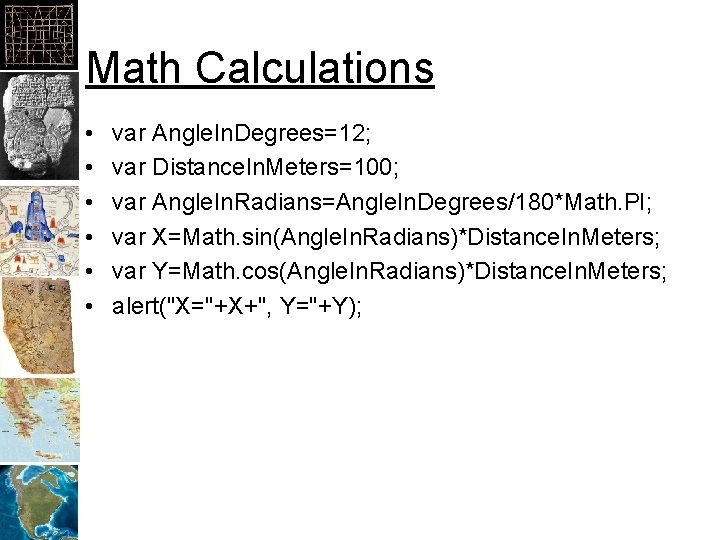Math Calculations • • • var Angle. In. Degrees=12; var Distance. In. Meters=100; var Angle. In. Radians=Angle. In. Degrees/180*Math. PI; var X=Math. sin(Angle. In. Radians)*Distance. In. Meters; var Y=Math. cos(Angle. In. Radians)*Distance. In. Meters; alert("X="+X+", Y="+Y);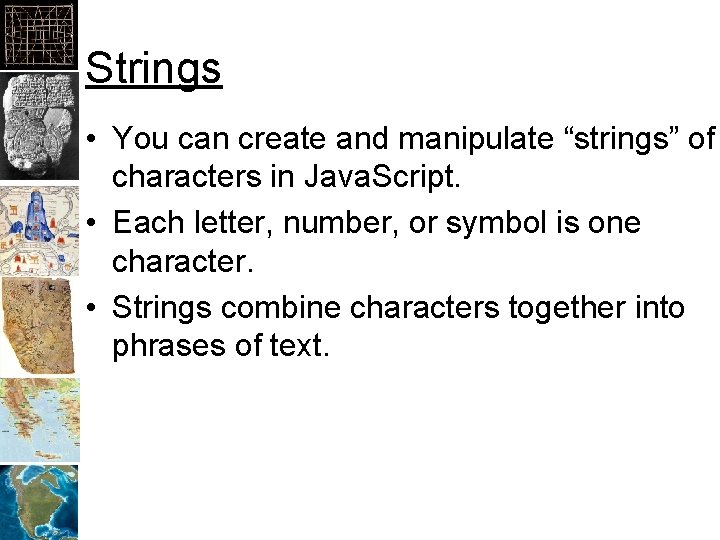Strings • You can create and manipulate “strings” of characters in Java. Script. • Each letter, number, or symbol is one character. • Strings combine characters together into phrases of text.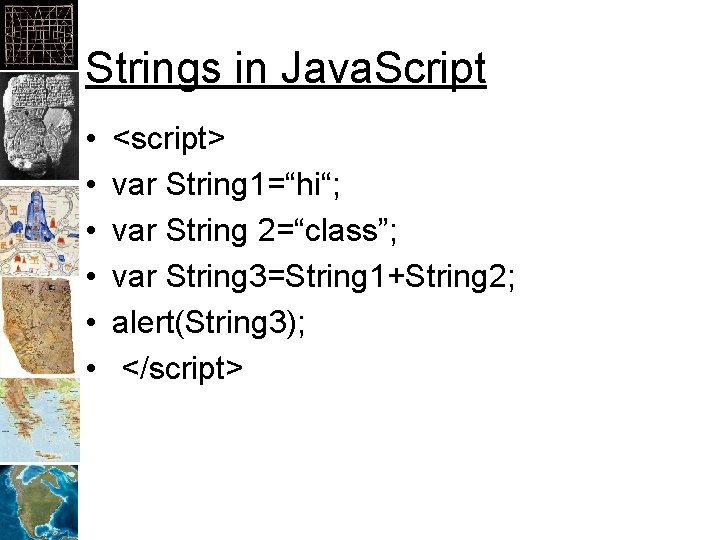Strings in Java. Script • • • <script> var String 1=“hi“; var String 2=“class”; var String 3=String 1+String 2; alert(String 3); </script>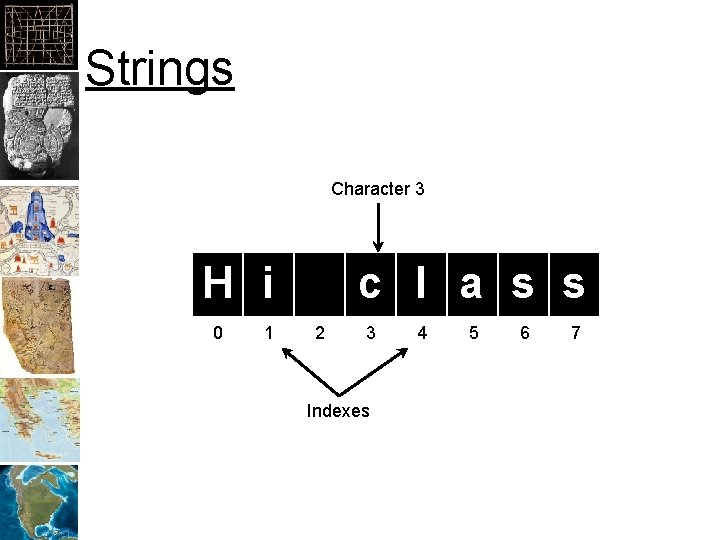Strings Character 3 H i 0 1 c l a s s 2 3 Indexes 4 5 6 7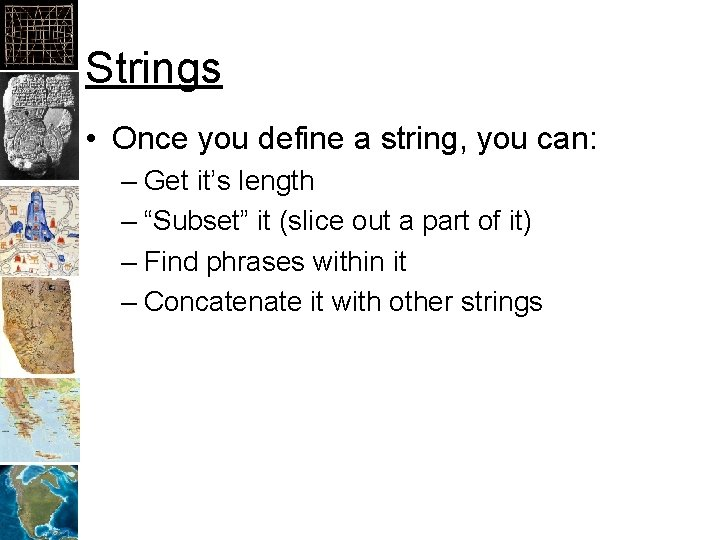Strings • Once you define a string, you can: – Get it’s length – “Subset” it (slice out a part of it) – Find phrases within it – Concatenate it with other stringsTypes • Variables in Java. Script are defined by a “type”. There are other types but the most common are: – Number – String • The type determines what you can do with it: – 1+1 = 2 – “ 1” + “ 1” = “ 11”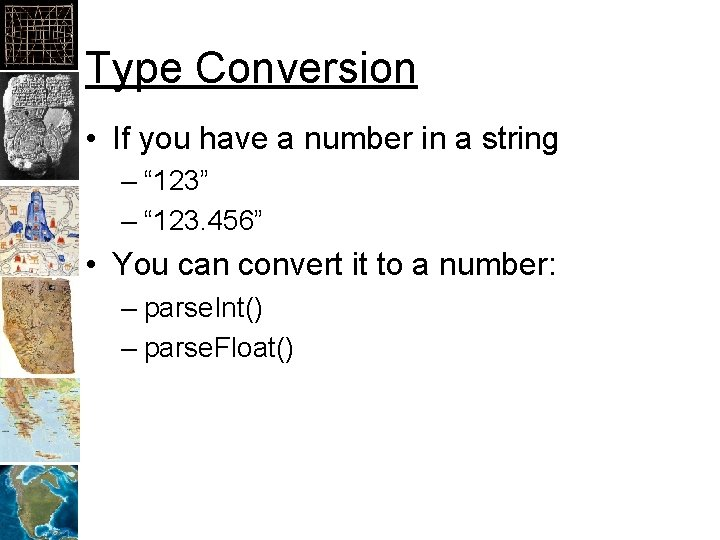Type Conversion • If you have a number in a string – “ 123” – “ 123. 456” • You can convert it to a number: – parse. Int() – parse. Float()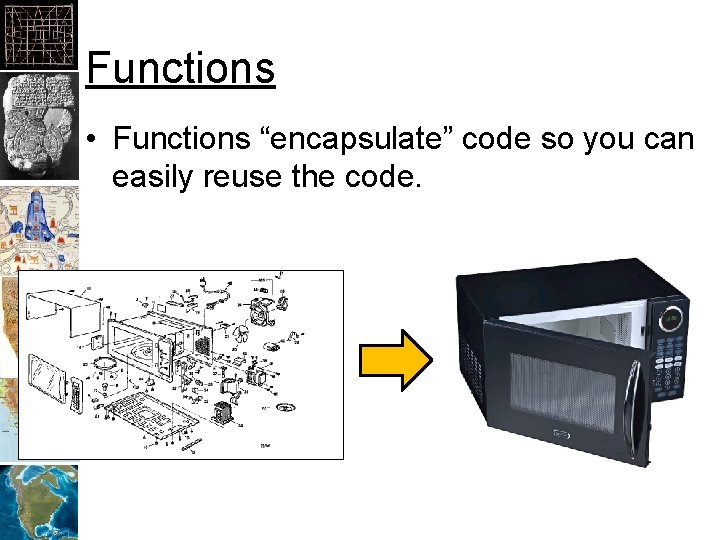Functions • Functions “encapsulate” code so you can easily reuse the code.Functions • • • • function Get. XFrom. Angle. And. Distance(Angle. In. Degrees, Distance. In. Meters) { var Angle. In. Radians=Angle. In. Degrees/180*Math. PI; var X=Math. sin(Angle. In. Radians)*Distance. In. Meters; return(X); } function Get. YFrom. Angle. And. Distance(Angle. In. Degrees, Distance. In. Meters) { var Angle. In. Radians=Angle. In. Degrees/180*Math. PI; var Y=Math. cos(Angle. In. Radians)*Distance. In. Meters; return(Y); } var Angle=10; var Distance=100; var X=Get. XFrom. Angle. And. Distance(Angle, Distance); var Y=Get. YFrom. Angle. And. Distance(Angle, Distance);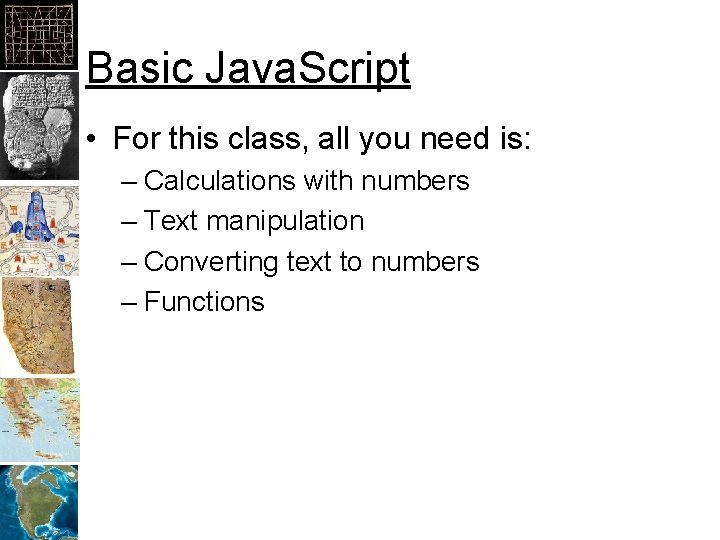Basic Java. Script • For this class, all you need is: – Calculations with numbers – Text manipulation – Converting text to numbers – Functions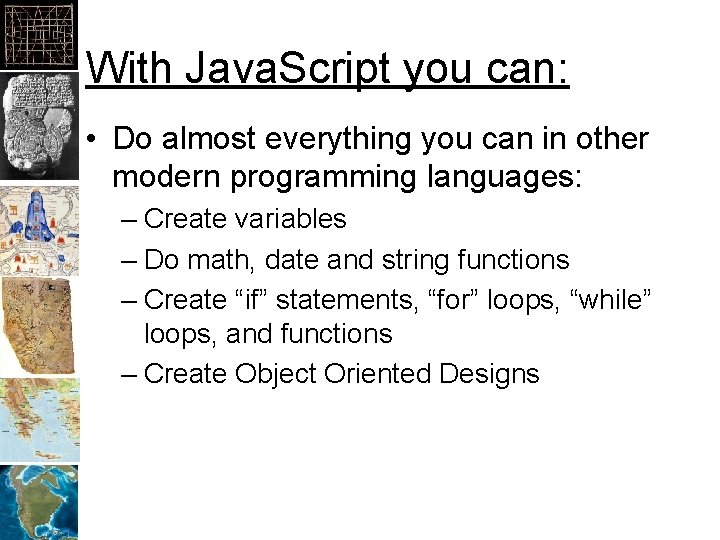With Java. Script you can: • Do almost everything you can in other modern programming languages: – Create variables – Do math, date and string functions – Create “if” statements, “for” loops, “while” loops, and functions – Create Object Oriented Designs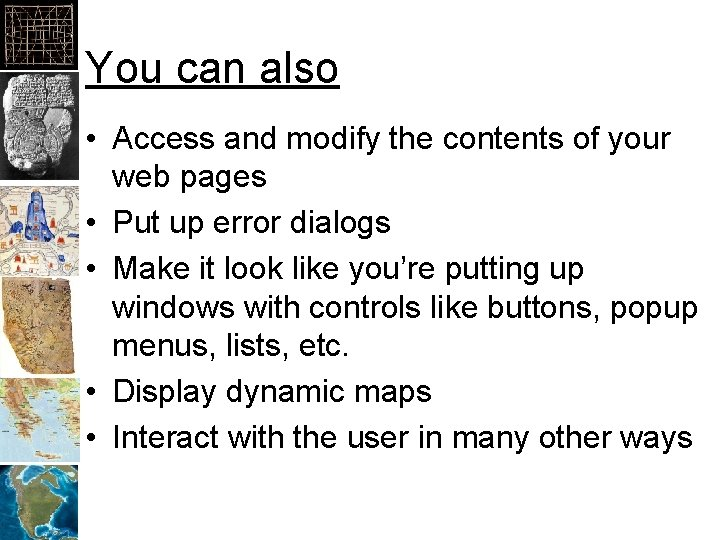You can also • Access and modify the contents of your web pages • Put up error dialogs • Make it look like you’re putting up windows with controls like buttons, popup menus, lists, etc. • Display dynamic maps • Interact with the user in many other waysJava. Script cannot • Access the local computers disk drive, network (other than it’s original server), printer, and other resources • Launch another program • Add viruses to your computer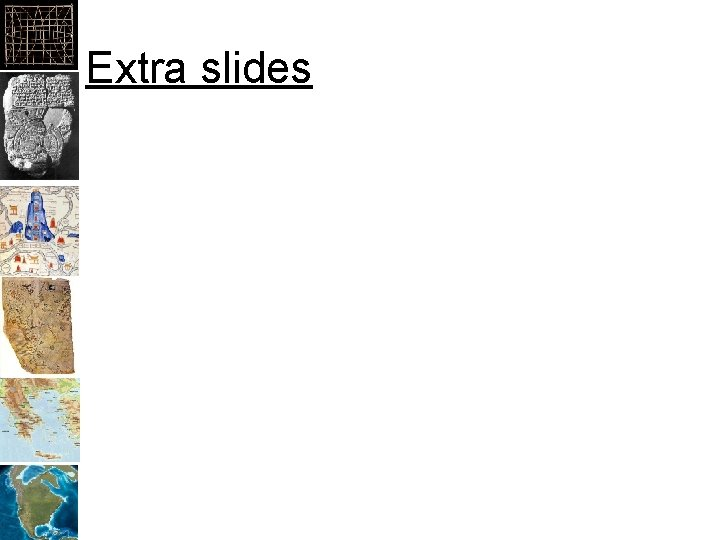Extra slides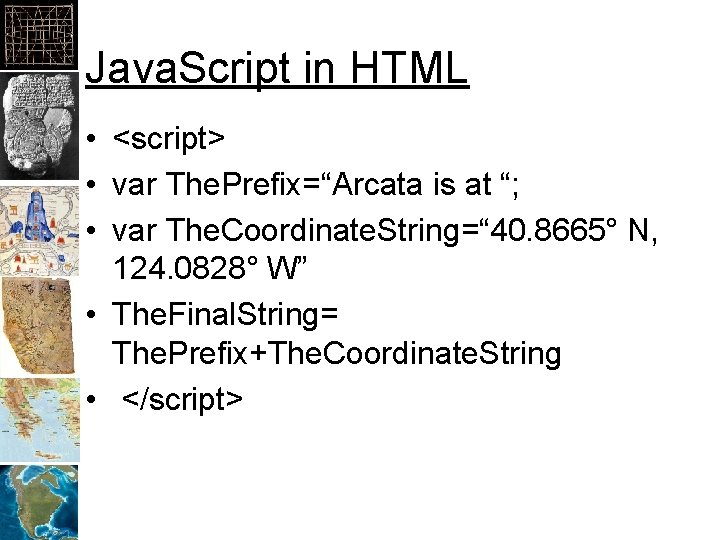Java. Script in HTML • <script> • var The. Prefix=“Arcata is at “; • var The. Coordinate. String=“ 40. 8665° N, 124. 0828° W” • The. Final. String= The. Prefix+The. Coordinate. String • </script>